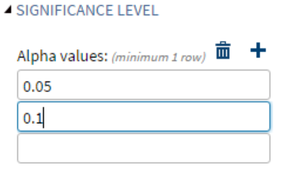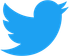Started ‎09-18-2020 by
Modified ‎08-03-2021 by
Views 4,339Next week, we will walk through a task that does power analysis for one-way anova. It will be an a large-scale modification of the built-in task for power analysis for pearson correlation. However, there are two elements of custom task development that you need to understand before we dive in that monster of a task. This week's post will help lay the ground work for next week. The first is a control called an Option Table, and the second is the Requirements portion of the VTL code. Both of which will be used several times in our task for allowing the user to provide as many values as they want.

### Option Tables

The OptionTable element allows you to organize options into columns. You can choose add the addRemoveRowTools attribute, which will allow the user to add and remove rows from the table. This comes in handy for our use in the power task because for Significance Levels, Sample Sizes, and Power Values, proc power allows the user to specify as many numbers as they want.

Here's an example of an option table from the built-in Pearson Correlation task:Here's the Metadata code for the Significance Level option table:

``````<Option inputType="string" name="alphaGroup">SIGNIFICANCE LEVEL</Option>
<Columns>
<Column defaultValues="0.05" label="Alpha" name="alpha">
<Option decimalPlaces="1,15" inputType="numbertext" invalidMessage="Enter a number for alpha that is greater than zero and less than 1" maxValue="0.99999999999" minValue="0.00000000001" missingMessage="Enter a number for alpha that is greater than zero and less than 1" promptMessage="Enter a number for alpha that is greater than zero and less than 1" rangeMessage="Enter a number for alpha that is greater than zero and less than 1" required="false"/>
</Column>
</Columns>
</OptionTable>  ``````

Here's the SAS Code to access the values in the Significance Level option table:

``````## Alpha
#set(\$_alpha=[])
#foreach(\$item in \$alphaTable.rows.values)
#if (\$item.alpha)
#end
#end
#if (\$_alpha.size() > 0) alpha=#foreach(\$item in \$_alpha) \$item#end#end``````

### Requirements

The requirements element allows you to specify a list of conditions (or requirements) that must be met before the task can be run. If the condition is false, a message is printed in green in the SAS Code window, and no code is generated. Each requirement has two pieces, the condition and the message. Conditions can be quickly become complex if your requirement is based on several controls in your task.

One common use of the requirements element is to require that one value be less than another value. This is helpful in situations where the user needs to specify a maximum and minimum. You do not want the code to generated if the user specifies a maximum that is less than the minimum.

Here's an example of a requirement from the Pearson Correlation Task that does just that:

``````<Requirement condition="!(             (\$powerBySampleSizePlot == '1') &amp;&amp;              (\$solveFor == 'solveforPowerChoice') &amp;&amp;              (\$usePlotMinSSValue == '1') &amp;&amp;              (\$usePlotMaxSSValue == '1') &amp;&amp;             (\$MathTool.toDouble(\$ssMinValue) &gt; \$MathTool.toDouble(\$ssMaxValue))         )">
<Message nlsKey="minMaxSampleSizePlotRequirementKey">The maximum sample size for the power by sample size plot cannot be less than the minimum value.</Message>
</Requirement>``````

### Join the conversation on Twitter! Use the hashtag #CustomTaskTuesday and tweet@OliviaJWright with your Custom Task comments and questions!